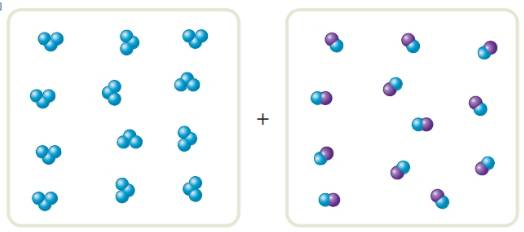Chapter 17, Problem 2ALQ### Introductory Chemistry: A Foundati...

9th Edition
Steven S. Zumdahl + 1 other
ISBN: 9781337399425

#### Solutions

Chapter
Section### Introductory Chemistry: A Foundati...

9th Edition
Steven S. Zumdahl + 1 other
ISBN: 9781337399425
Textbook Problem
1 views

# The boxes shown below represent a set of initial conditions for the reaction:Draw a quantitative molecular picture that shows what this system looks like after the reactants are mixed in one of the boxes and the system reaches equilibrium. Support your answer with calculations.Consider an equilibrium mixture of four chemicals (A, B, C, and D, all gases) reacting in a closed flask according to the foll owing equation: A + B ⇌ C + D a. You add more A to the flask. How does the concentration of each chemical compare to its original concentration after equilibrium is re-established? Justify your answer.b. You have the original set-up at equilibrium, and add more D to the flask. How does the concentration of each chemical compare to its original concentration after equilibrium is re-established? Justify your answer.

Interpretation Introduction

Interpretation:

The quantitative molecular picture that shows that this system looks like after the reactants are mixed in one of the boxes and the system reaches equilibrium is to be drawn.

Concept Introduction:

In a chemical equilibrium the concentration of the reactants and products remain constant. The rate of the forward reaction is equal to the rate of backward reaction.

Explanation

The given reaction is,

The quantitative molecular picture that shows that this system looks like after the reactants are mixed in one of the boxes and the system reaches equilibrium is,

Figure 1

 A 8 5 3 3

### Still sussing out bartleby?

Check out a sample textbook solution.

See a sample solution

#### The Solution to Your Study Problems

Bartleby provides explanations to thousands of textbook problems written by our experts, many with advanced degrees!

Get Started

#### Find more solutions based on key concepts